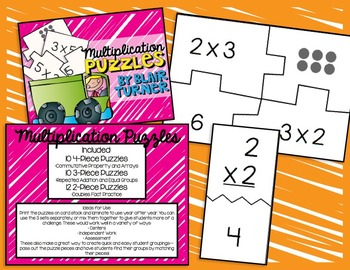# Math Puzzle Pack - Place Value, Addition, Multiplication, and Fractions!2nd - 4th
Subjects
Standards
Resource Type
Formats Included
• Zip
Pages
96 pages

### Description

This is a BUNDLED pack of 4 of my place value, addition, multiplication, and fractions puzzles. This is perfect for a resource room, intervention, or inclusion teacher - or any classroom teacher looking to help reinforce skills at multiple levels.

You will get FOUR separate puzzle products in a zipped file. That's 96 pages worth of puzzles! By buying them together, you are getting 4 products for the price of 3.

Included in this Puzzle Pack:

Fraction Puzzles - Whole, Set, and Number Line Models

Included:

34 4-Piece Fraction Puzzles (2 puzzles per page)
-Pieces include: fraction in standard form, fraction of a whole model, fraction of a set model, fraction on a number line model
-Puzzles include halves, thirds, fourths, fifths, sixths, and eighths

CCSS.Math.Content.3.NF.A.1
CCSS.Math.Content.3.NF.A.2

Multiplication Puzzles - Common Core Aligned

Included are 3 sets of puzzles:

-10 4-piece puzzles
-Commutative property, arrays
-CCSS.Math.Content.3.OA.A.1
CCSS.Math.Content.3.OA.B.5

-10 3-piece puzzles
-Repeated addition sentences, equal groups models
-CCSS.Math.Content.3.OA.C.7

-12 2-piece puzzles
-Double multiplication facts
-CCSS.Math.Content.3.OA.A.1
CCSS.Math.Content.3.OA.A.3

Addition Puzzles - Common Core Aligned

Reinforce addition concepts with these fun, engaging, and self-checking puzzles! These puzzles are aligned with 1st & 2nd grade Common Core standards for addition, but could also be used with older students who still need to work on these skills.

Included are 3 sets of puzzles:

-10 4-piece puzzles
-CCSS.Math.Content.1.NBT.C.4
-CCSS.Math.Content.2.OA.B.2

-10 3-piece puzzles
-Decomposing Numbers 11-19
-CCSS.Math.Content.1.NBT.B.2b
-CCSS.Math.Content.2.OA.B.2

-12 2-piece puzzles
-CCSS.Math.Content.1.NBT.C.4
-CCSS.Math.Content.2.OA.B.2

Place Value Puzzles - Standard, Expanded, and Word Form

Included are 3 sets of puzzles:
10 2-Digit Puzzles
10 3-Digit Puzzles
10 4-Digit Puzzles

Aligned with Common Core Standards:

CCSS.Math.Content.2.NBT.A.1
CCSS.Math.Content.2.NBT.A.3

Print the puzzles on card stock and laminate to use year after year. Mix them up and let students get started matching the fraction models!

These are a great addition to your math centers--students can use them independently and they can be used over and over!

Total Pages
96 pages
N/A
Teaching Duration
N/A
Report this Resource to TpT
Reported resources will be reviewed by our team. Report this resource to let us know if this resource violates TpT’s content guidelines.

### Standards

to see state-specific standards (only available in the US).
Fluently add and subtract within 20 using mental strategies. By end of Grade 2, know from memory all sums of two one-digit numbers.
Interpret products of whole numbers, e.g., interpret 5 × 7 as the total number of objects in 5 groups of 7 objects each. For example, describe a context in which a total number of objects can be expressed as 5 × 7.
Use multiplication and division within 100 to solve word problems in situations involving equal groups, arrays, and measurement quantities, e.g., by using drawings and equations with a symbol for the unknown number to represent the problem.
Apply properties of operations as strategies to multiply and divide. Examples: If 6 × 4 = 24 is known, then 4 × 6 = 24 is also known. (Commutative property of multiplication.) 3 × 5 × 2 can be found by 3 × 5 = 15, then 15 × 2 = 30, or by 5 × 2 = 10, then 3 × 10 = 30. (Associative property of multiplication.) Knowing that 8 × 5 = 40 and 8 × 2 = 16, one can find 8 × 7 as 8 × (5 + 2) = (8 × 5) + (8 × 2) = 40 + 16 = 56. (Distributive property.)
Fluently multiply and divide within 100, using strategies such as the relationship between multiplication and division (e.g., knowing that 8 × 5 = 40, one knows 40 ÷ 5 = 8) or properties of operations. By the end of Grade 3, know from memory all products of two one-digit numbers.